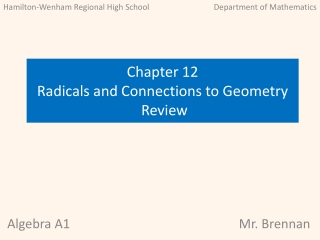Download PresentationAlgebra A1

# Algebra A1

Télécharger la présentation## Algebra A1

- - - - - - - - - - - - - - - - - - - - - - - - - - - E N D - - - - - - - - - - - - - - - - - - - - - - - - - - -
##### Presentation Transcript

1. Hamilton-Wenham Regional High School Department of Mathematics Chapter 12 Radicals and Connections to Geometry Review Algebra A1 Mr. Brennan

2. Hamilton-Wenham Regional High School Department of Mathematics Chapter 12 Review Radicals and Connections to Geometry Learning Objectives: Chapter 12 Algebra A1 Mr. Brennan

3. Hamilton-Wenham Regional High School Department of Mathematics Chapter 12 Review Radicals and Connections to Geometry Learning Objectives: Chapter 12 Algebra A1 Mr. Brennan

4. Chapter 12 Radicals and Connections to Geometry The review for chapter 12 has three types of slides • Review material with goals and definitions • Examples with solutions (22) • Practice problems There are 57 practice problems for Chapter 12. Work out the problems as you encounter them. The answer to each question is displayed after each question.

5. Chapter 12 Quick Links 12.1 Functions Involving Square Roots 12.2 Operations with Radical Expressions 12.3 Solving Radical Expressions 12.4 Completing the Square 12.5 The Pythagorean Theorem and Its Converse 12.6 The Distance and Midpoint Formulas 12.7 Trigonometric Ratios: Exploring Data and Statistics 12.8 Logical Reasoning: Proof

6. Review Functions Involving Square Roots Lesson 12.1

7. Examples Solution

8. Practice Solution

9. Examples Solution

10. Practice Solution

11. Examples Solution

12. Practice Solution

13. Review Operations with Radical Expressions Lesson 12.2

14. Examples Solution

15. Practice Solution

16. Examples Solution

17. Practice Solution

18. Examples Solution

19. Practice Solution

20. Examples Solution

21. Practice Solution

22. Review Solving Radical Expressions Lesson 12.3

23. Examples Solution

24. Practice Solution

25. Examples Solution

26. Practice Solution

27. Examples Solution

28. Practice Solution

29. Review Completing the Square Lesson 12.4

30. Examples Solution

31. Practice Solution

32. Examples Solution

33. Practice Solution These are sample answers: Choose a method to solve the quadratic equation. Explain your choice. 4. finding square roots because the equation is of the form 5. factoring because the quadratic can be easily factored 6. Using quadratic formula because the quadratic contains decimals.

34. Review The Pythagorean Theorem and Its Converse Lesson 12.5

35. Examples Solution

36. Practice Solution

37. Examples Solution

38. Practice Determine whether the given lengths are sides of a right triangle. Solution

39. Review The Distance and Midpoint Formulas Lesson 12.6

40. Examples Solution

41. Practice Solution Find the distance between the two points. Round the result to the nearest hundredth if necessary.

42. Examples Solution

43. Practice Solution

44. Examples Solution

45. Practice Solution

46. Examples Solution

47. Practice Solution

48. Review Trigonometric Ratios: Exploring Data and Statistics Lesson 12.7

49. Examples Solution

50. Practice Solution